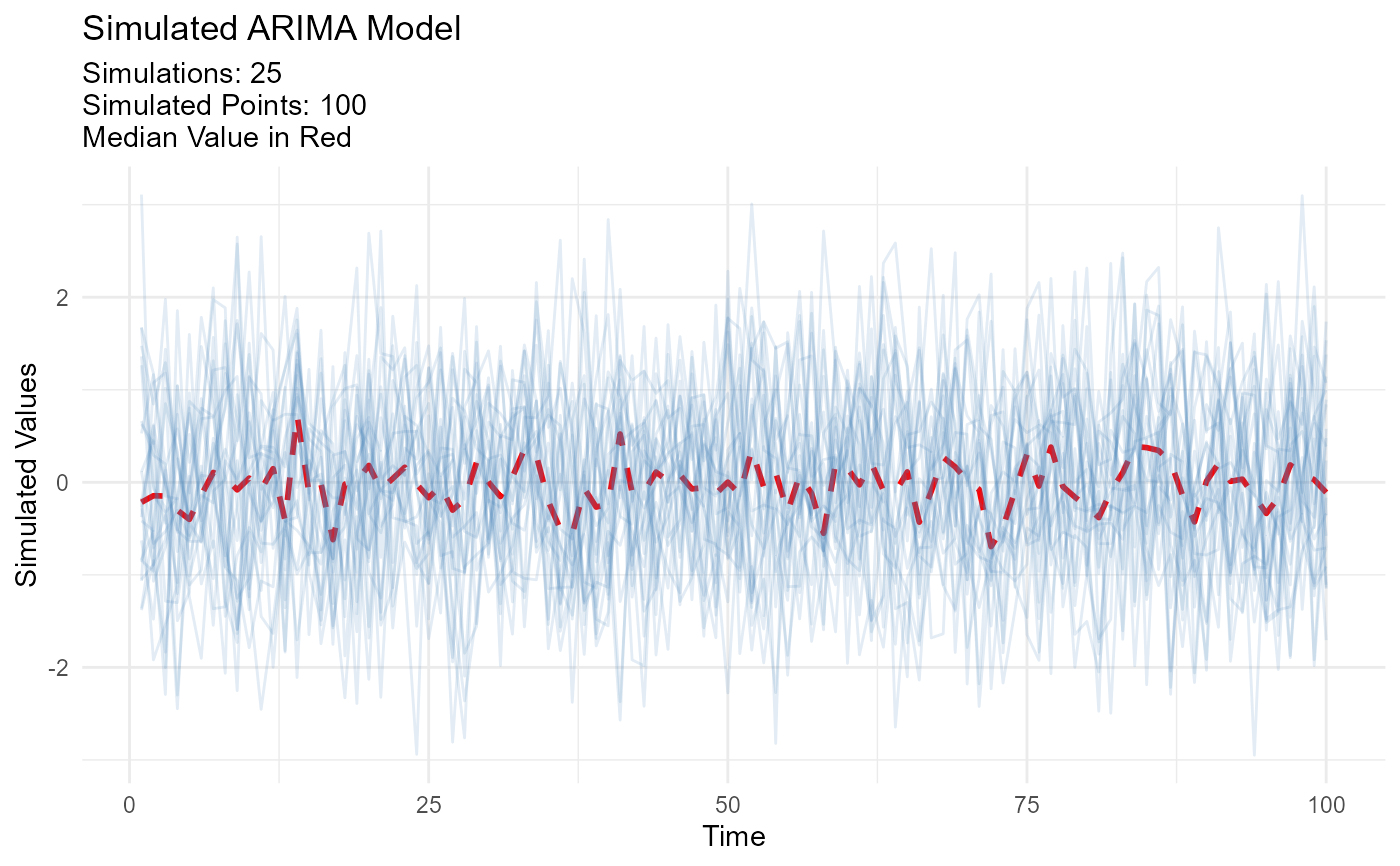Returns a list output of any n simulations of a user specified ARIMA model. The function returns a list object with two sections:

• data

• plots

The data section of the output contains the following:

• simulation_time_series object (ts format)

• simulation_time_series_output (mts format)

• simulations_tbl (simulation_time_series_object in a tibble)

• simulations_median_value_tbl (contains the stats::median() value of the simulated data)

The plots section of the output contains the following:

• static_plot The ggplot2 plot

• plotly_plot The plotly plot

## Usage

ts_arima_simulator(
.n = 100,
.num_sims = 25,
.order_p = 0,
.order_d = 0,
.order_q = 0,
.ma = c(),
.ar = c(),
.sim_color = "steelblue",
.alpha = 0.05,
.size = 1,
...
)

## Arguments

.n

The number of points to be simulated.

.num_sims

The number of different simulations to be run.

.order_p

The p value, the order of the AR term.

.order_d

The d value, the number of differencing to make the series stationary

.order_q

The q value, the order of the MA term.

.ma

You can list the MA terms respectively if desired.

.ar

You can list the AR terms respectively if desired.

.sim_color

The color of the lines for the simulated series.

.alpha

The alpha component of the ggplot2 and plotly lines.

.size

The size of the median line for the ggplot2

...

Any other additional arguments for stats::arima.sim

A list object.

## Details

This function takes in a user specified arima model. The specification is passed to stats::arima.sim()

output <- ts_arima_simulator()
output$plots$static_plot